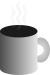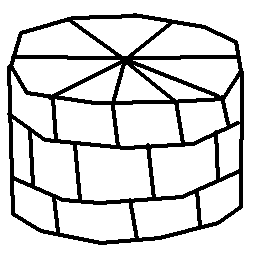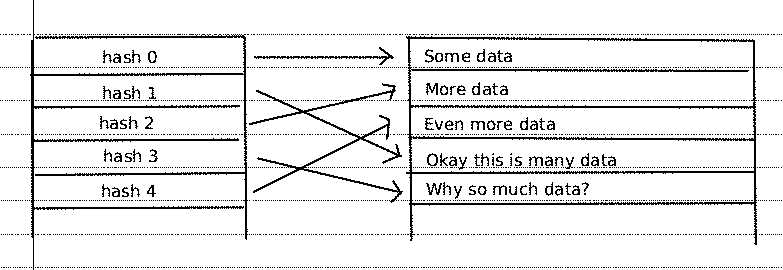#Coffee SpaceListen:

## Database ConceptPreview Image

For the dead social project the 'database' is just a series of files on disk, which worked initially, but there are now some 4k posts (at the time of writing) and 6.4k tags, each of which represent a single file.

For a PhD project I ran over a million experiments in a directory, each of which generated their own log file. This essentially broke the EXT partition and the drive later died!

Clearly, what I need is a simple, performant, flat file database.

After the relative success of writing uServer, I am wondering whether I could write something similar but for databases.

### Requirements

Ideally this is all to be achieved within the 256 line limit (with comments), as was seen with uServer.

I would have the following basic requirements of the interface:

• Add data with some key (ID).
• Retrieve data with some key (ID).
• Edit data with some key (ID).
• Delete data with some key (ID). (Note: This one is less important as dead social never really deletes anything.)

And the following requirements of the operations:

• Fast - It's no good if it's not fast - it needs to be super speedy.
• Detect duplicate keys - We don't want to accidentally override data, so it better be damn sure it knows what it's doing.
• Flat file structure - Of course we want to address the issue with the original database.

What we do not expect, but would be nice to have:

• Caching - Ideally we limit the disk thrashing where possible.
• Thread safety - It would be great if it could be accessed by multiple threads, but this might be too much to ask for in such a small library.

### Existing

I quite like the simplicity of Google's LevelDB, where it essentially offers the methods:

• The basic operations are `Put(key,value)`, `Get(key)`, `Delete(key)`.

It also offers tonnes of other features too.

### Structure

One important consideration is the ability to read/write files really fast. Ideally this is done with an $O\left(1\right)$ look-up where possible. We look to achieve this with the following data structure:Proposed database structure

#### Hash Map Table

The first structure is the hash map table. This file would look approximately like so:

`0001 [small_hash]: [data_index]`

We would have some hash function `H()` take a `key` string and produce a hash key `k`. A small version of this would look at an index in the lookup array and point to the data file.

Our key is of size ${K}_{bits}$ and we round this to the closest number of bytes ${K}_{bytes}$. The size of the pre-built look-up table is therefore ${K}_{bytes}×{2}^{{K}_{bits}}$. Here is some example of table sizes:

${k}_{bits}$ ${K}_{bytes}$ Equation Result (bytes) Results
$8$ $1$ $1×{2}^{8}$ 256 256B
$10$ $2$ $2×{2}^{10}$ 2,048 2kB
$16$ $2$ $2×{2}^{16}$ 131,072 128kB
$24$ $3$ $3×{2}^{24}$ 50,331,648 48MB
$32$ $4$ $4×{2}^{32}$ 17,179,869,184 16GB
$64$ $8$ $8×{2}^{64}$ 147,573,952,589,676,412,928 134.2EB

So this method is fine for small hash bit values, but doesn't scale well as the number of bits are increased. Generally we will try to keep our hash keys under 24 bits and handle hash collisions as they occur. This allows for a maximum of ${2}^{24}$ entries (approximately 16 million entries), which shouldn't cause too many issues up until approximately 1 million entries or so.

We can do a little better though. If we checkout our original equation ${K}_{bytes}×{2}^{{K}_{bits}}$, we can actually reinterpret this as ${M}_{bytes}×{2}^{{K}_{bits}}$, where ${M}_{bytes}$ are the number of bytes used for lookup. We can therefore have a maximum of ${2}^{\left(}8×{M}_{bytes}\right)$ entries. Some example sizes:

${k}_{bits}$ ${M}_{bytes}$ Equation Result (bytes) Results
$32$ $3$ $3×{2}^{32}$ 12884901888 12GB
$16$ $3$ $3×{2}^{16}$ 196608 192kB

Note: It doesn't make sense for ${2}^{8×{M}_{bytes}}>{K}_{bits}$, as it means you have a larger address space than you can actually address.

If we are willing to break our $O\left(1\right)$ requirement, we can actually instead use the mapping as a search accelerator, rather than a proper mapping. Instead of the hashed key $k$ directly reporting an index in the database, it could instead suggest that the data can be found at a multiple of the given index. This way we can reduce the value of ${M}_{bytes}$, where a value of 2 would indicate we check the same index offset every ${2}^{\left(}8×2\right)$ records at a small cost of search time. This would also require that each record contain the full hash size to ensure the correct entry was found.

Another hash map table structure could be:

`0002 [small_hash]: [large_hash] [data_index]`

Where we loop through offsets of `small_hash` until we find a match for the `large_hash`. This would also allow for the hash table to increase in size as more data is added at a small cost of searching - this time inside the hash table rather than the data table.

Note: It may also be worth considering building the hash table in RAM for speed by doing an initial parse of the database during initialisation - rather than keeping it on disk. If `small_hash` is derived from `large_hash` then we would only need the database file in order to rebuild the hash map table.

#### Database

The database entries on the other hand would likely be quite boring, with a structure such as:

`0003 [data_index]: [large_hash] [value]`

Note: It might also be worth considering checking for data integrity.

### Interface

An interface may look as follows:

```0004 /**
0005  * udatabase_create()
0006  *
0007  * Create a database on disk.
0008  *
0009  * @param filename The location of the database to be create.
0010  * @return Creation success result.
0011  **/
0012 int udatabase_create(char* filename);
0013
0014 /**
0015  * udatabase_init()
0016  *
0017  * Open a database for read/write operations.
0018  *
0019  * @param filename The location of the database to be operated on.
0020  * @param size The maximum size of a stored item.
0021  * @param cache Number of items to store in the cache.
0022  * @return Initialisation success result.
0023  **/
0024 int udatabase_init(char* filename, int size, int cache);
0025
0026 /**
0027  * udatabase_shash()
0028  *
0029  * Convert a string to a hash.
0030  *
0031  * @param s The NULL terminated string to be hashed.
0032  * @param b The number of bits to hash the string to.
0033  * @return The hashed number.
0034  **/
0035 /* NOTE: https://gitlab.com/danbarry16/u-server/-/commit/5ebd673a2b96b8c2e1897cd1c733e6857902b904 */
0036 /* NOTE: https://stackoverflow.com/questions/7666509/hash-function-for-string */
0037 unsigned int udatabase_shash(char* s, unsigned int b);
0038
0039 /**
0040  * udatabase_scmp()
0041  *
0042  * Compare two NULL terminated strings, checking if equal.
0043  *
0044  * @param a The first string to be checked.
0045  * @param b The substring to be checked that is NULL terminated.
0046  * @param c Treat as a NULL character in string a.
0047  * @return A pointer to the end of the match if equal, otherwise NULL.
0048  **/
0049 /* NOTE: Check userver_scmp(). */
0050 char* udatabase_scmp(char* a, char* b, char c);
0051
0052 /**
0053  * udatabase_put()
0054  *
0055  * Put data into the database using the given key. It does not check if the key
0057  *
0058  * @param k A NULL terminated key string to be used to insert a value.
0059  * @param v A NULL terminated value string to be inserted into the database.
0060  * @return The success of the insertion.
0061  **/
0062 int udatabase_put(char* k, char* v);
0063
0064 /**
0065  * udatabase_get()
0066  *
0067  * Get the data stored at the location if it exists.
0068  *
0069  * @param k A NULL terminated key string value to be searched for.
0070  * @return The value associated with the key, otherwise NULL.
0071  **/
0072 char* udatabase_get(char* k);
0073
0074 /**
0075  * udatabase_close()
0076  *
0077  * Close the database so that no further operations can be performed.
0078  *
0079  * @return Tne success of closing.
0080  **/
0081 int udatabase_close();```

We should be able to make use of `fseek()` in order to read and write at given offsets, without needs to crawl the entire flat binary.

With regards to reading/writing structured data, using something like JSON would be nice in theory, but actually a pain to write simply and concisely. Instead I could imagine writing something like follows:

```0082 struct Post{
0083   char type;
0084   unsigned long id;
0086 ];
0087
0088 char* buff = udatabase_get("some key");
0089 if(buff != NULL){
0090   /* Make sure the structured data is of the type we expect */
0091   if(buff == 'p'){
0092     struct Post* post = (struct Post*)buff;
0093     /* TODO: Rest of code here on structured data. */
0094   }else{
0095     /* TODO: Unknown data format. */
0096   }
0097 }else{
0098   /* TODO: Handle read error. */
0099 }```

Maybe another update in a few weeks when I iron out the details (and get some time).